# 4.8: Conformations of Disubstituted Cyclohexanes

Objective

After completing this section, you should be able to use conformational analysis to determine the most stable conformation of a given disubstituted cyclohexane.

Key Terms

Make certain that you can define, and use in context, the key term below.

• conformational analysis

Study Notes

When faced with the problem of trying to decide which of two conformers of a given disubstituted cyclohexane is the more stable, you may find the following generalizations helpful.

1. A diequatorial conformation will always be more stable than a diaxial one.
2. When one substituent is axial and the other is equatorial, the most stable conformation will be the one with the bulkiest substituent in the equatorial position. Steric bulk decreases in the order

tert-butyl > isopropyl > ethyl > methyl > hydroxyl > halogens

## Monosubstituted Cyclohexanes

The conformation in which the methyl group is equatorial is more stable, and thus the equilibrium lies in this direction.The relative steric hindrance experienced by different substituent groups oriented in an axial versus equatorial location on cyclohexane may be determined by the conformational equilibrium of the compound. The corresponding equilibrium constant is related to the energy difference between the conformers, and collecting such data allows us to evaluate the relative tendency of substituents to exist in an equatorial or axial location. Table $$\PageIndex{1}$$ show some of these free energy values (sometimes referred to as A values).

Table: $$\PageIndex{1}$$: Free Energy Differences for Cyclohexyl Substituents
Substituent Group ΔG º (axial–equatorial) Substituent Group ΔG º (axial–equatorial)
CH3 1.74 kcal mol-1 F 0.3
C2H5 1.8 Cl 0.6
(CH3)2CH 2.2 Br 0.6
(CH3)3C 4.7 I 0.55
CF3 2.4 OH 0.6 - 1.0
C6H5 2.8 OCH3 0.65
CH2=CH 1.6 OCOCH3 0.75
HC≡C 0.45 OSI(CH3)3 0.7
CN 0.2 SH 1.2
CHO 0.7 NH2 1.3 - 1.7
COCH3 1.2 N(CH3)2 2.0
CO2H 1.4 N3 0.5
CO2 Na 2.0 NO2 1.1
CO2CH3 1.3 Si(CH3)3 2.5
COCl 1.3
These "Conformational Energies" or "A values" are -ΔG° 's for axial/equatorial equilibria of substituted cyclohexanes. The data in this table are averaged from several different sources. For a more precise and extensive compilation see: E.L.Eliel and S.H.Wilen, Stereochemistry of Organic Compounds, 1994.

Looking at the energy values in Table $$\PageIndex{1}$$, it is clear that the apparent "size" of a substituent (in terms of its preference for equatorial over axial orientation) is influenced by its width and bond length to cyclohexane, as evidenced by the fact that an axial vinyl group is less hindered than ethyl, and iodine slightly less than chlorine.

We noted earlier that cycloalkanes having two or more substituents on different ring carbon atoms exist as a pair (sometimes more) of configurational stereoisomers. Now we must examine the way in which favorable ring conformations influence the properties of the configurational isomers. Remember, configurational stereoisomers are stable and do not easily interconvert, whereas, conformational isomers normally interconvert rapidly. In examining possible structures for substituted cyclohexanes, it is useful to follow two principles:

1. Chair conformations are generally more stable than other possibilities.
2. Substituents on chair conformers prefer to occupy equatorial positions due to the increased steric hindrance of axial locations.

## Dichlorocyclohexanes

The next level of complexity are di-substituted cycloalkanes and we will use dichlorocyclohexane as the model system for discussing these compounds. The first question we must ask is which C the two chlorine substituents are on. For now, I want to discuss 1,3- dichlorocyclohexane. This introduces another issue: are the two Cl on the same side of the ring, or on opposite sides? We call these "cis" (same side) and "trans" (opposite sides). We focus on one of these, cis-1,3-dichlorocyclohexane. And for now, we will just look at hexagon structural formulas, leaving the question of conformation for later. Let's go through this one step at a time.

### 1,3-Dichlorocyclohexane

Our first attempt to draw 1,3-dichlorocyclohexane might look something like Figure $$\PageIndex{1}$$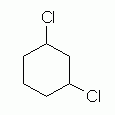Figure $$\PageIndex{1}$$

The structure in Figure 4.8.1 is indeed a dichlorocyclohexane. It is even a 1,3-dichlorocyclohexane. However, this structure provides no information about the orientation of the two Cl atoms relative to the plane of the ring. To show a specific isomer -- cis or trans -- we must somehow show how the two Cl atoms are oriented relative to the plane of the ring.

### cis-1,3-Dichlorocyclohexane

Figure 4.8.2 shows two common ways to show how the substituents are oriented relative to the plane of the ring. The compound shown here is cis-1,3-dichlorocyclohexane.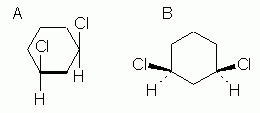Figure $$\PageIndex{2}$$

The basic idea in both of these is that we can imagine the ring to be planar, and then show the groups above or below the plane of the ring. How we show this is different in the two parts of Figure $$\PageIndex{2}$$. In Figure $$\PageIndex{1A}$$, we look at the ring "edge-on". The thick line for the bottom bond is intended to convey the edge-on view (or side view); this is sometimes omitted, especially with hand-drawn structures, but be careful then that the meaning is clear. Once we understand that we are now looking at the ring edge-on, it is clear that the two Cl atoms are both above the ring, hence cis. In Figure $$\PageIndex{2B}$$, we view the ring "face-on" (or top view), and use special bond symbols -- "stereo bonds" -- to convey up and down: the heavy wedge -- an "up wedge" -- points upward, toward you, and the dashed bond -- a "down bond" -- points downward, away from you. Again, both Cl are "up", hence cis.

Note

For some notes on how to draw the stereo bonds, see the section E.1. Note: How to draw stereo bonds ("up" and "down" bonds).

In discussing Figure $$\PageIndex{2}$$, I started by saying that we imagine the ring to be planar. Emphasize that cycloalkane rings are not really planar (except for cyclopropane rings). As so often, the structural formula represents the general layout of the atoms, but not the actual molecular geometry.

### The conformation of cis-1,3-dichlorocyclohexane

Figure $$\PageIndex{3}$$, at the right, is one way to show this. Figure 4.8.3 shows features of the compound that we have already noted -- plus one more. Let's go through these features, emphasizing what is new.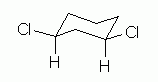Figure $$\PageIndex{3}$$

The structure shows cis-1,3-dichlorocyclohexane: a 6-ring; 2 Cl atoms, at positions 1 and 3; and cis, with both Cl on the same side of -- above -- the H that is on the same C.

I showed the 2 Cl atoms at corner positions, and I showed the H at the key positions explicitly. These points follow from some of the Helpful Hints discussed earlier. It is not required that you do these things, but they can make things easier for you -- and for anyone reading your structures.

Now, what is new here? The conformation. We start with the notion that the conformation of cyclohexane derivatives is based on the "chair". At each position, one substituent is axial (loosely, perpendicular to the ring), and one is equatorial (loosely, in the plane of the ring). There is more room in the equatorial positions (not easily seen with these simple drawings, but ordinary ball and stick models do help with this point). Thus we try to put the larger substituents in the equatorial positions. In this case, we put the Cl equatorial and the H axial at each position 1 and position 3.

We are now done with this compound, cis-1,3-dichlorocyclohexane. However, we have missed one very important concern -- because it is not an issue in this case. So let's look at another compound.

## cis-1,2-Dichlorocyclohexane

cis-1,2-Dichlorocyclohexane is like the previous compound, except that the two chloro groups are now at 1,2, rather than at 1,3. Figure 4.8.4 shows a simple structural formula for 1,2-dichlorocyclohexane, without orientation. This is analogous to Figure $$\PageIndex{1}$$ for the 1,3 isomer.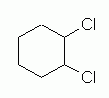Figure $$\PageIndex{4}$$: 1,2-dichlorocyclohexane: simple hexagon, with no info about orientation.

Figure 4.8.5 shows two ways to show the cis orientation in cis-1,2-dichlorocyclohexane. This Figure is analogous to Figure 4.8.3 above for the 1,3 isomer.Figure $$\PageIndex{15}$$

Figures $$\PageIndex{4}$$ and $$\PageIndex{5}$$ introduce no new ideas or complications; these two figures should be straightforward. So, what is the preferred conformation of cis-1,2-dichlorocyclohexane? This requires careful consideration; an important lesson from this exercise is to realize that we cannot propose a good conformation based simply on what we have learned so far. The two guidelines we have so far for conformation of 6-rings are:

• The carbon ring is in a chair.
• Larger substituents are in equatorial positions.

Let's explore the difficulty here by looking at some things people might naively draw. Figure $$\PageIndex{6}$$ shows an attempt to draw a chair conformation of cis-1,2-dichlorocyclohexane. It satisfies both of the guidelines listed above. But it is wrong.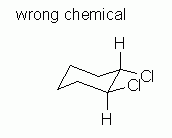Figure $$\PageIndex{6}$$: An attempt to draw a chair conformation of cis-1,2-dichlorocyclohexane. But this is the wrong chemical.

Why is Figure $$\PageIndex{6}$$ wrong? It is the wrong chemical. The structure shown in Figure $$\PageIndex{6}$$ is trans, not cis. Look carefully at the 1 and 2 positions. At one of them, the H is above the Cl; at the other, the Cl is above the H. Trans. Wrong chemical. The structure shown in Figure $$\PageIndex{6}$$ is not the requested chemical. Figure $$\PageIndex{7}$$ attempts to fix the problem with Figure $$\PageIndex{6}$$. However, it is also wrong.Figure $$\PageIndex{7}$$

Why is Figure $$\PageIndex{8}$$ wrong? After all, it seems to address the criteria presented. It contains both of the larger atoms (Cl) equatorial, and they are cis as desired. However, in Figure 4.8.7, the two axial groups on carbons # 1 and 2 (the two H that are shown) are both pointing up. This is impossible. In a valid chair, the axial groups alternate up/down as one goes around the ringFigure $$\PageIndex{8}$$

This follows from the tetrahedral bonding of C. Adjacent axial groups, as relevant here, must point in opposite directions; that condition is violated here. That is, Figure $$\PageIndex{7}$$ is not a valid chair.

So now what? We have a contradiction. And that really is the most important point here. It is important to realize that we cannot draw a conformation for cis-1,2-dichlorocyclohexane which easily fits the criteria we have used so far: a chair, with large groups equatorial. So what do we do? Clearly, the conformation of this compound must, in some way, involve more issues than what we have considered so far.

Instructors and books will vary in how much further explanation they want to give on this matter. Therefore, how you proceed from here must take into account the preferences in your course. Here is one way to proceed.

One simple way to proceed is to re-examine the two criteria we have been using, and then state a generality about what to do in the event of a conflict. That generality is: use the chair, and then fit the groups as best you can. That is, try to put as many of the larger groups equatorial as you can, but realize that you may not get them all equatorial.Figure $$\PageIndex{9}$$: A plausible chair conformation of cis-1,2-dichlorocyclohexane.

What have we accomplished here?

• First, this is the correct compound. Convince yourself that this really is cis-1,2-dichlorocyclohexane. In particular, it is cis because at each substituted position the Cl is "above" the H; that is, both Cl are on the same side of the ring.
• Second, this is a proper chair. Adjacent axial groups point in opposite directions. Only two of them are shown here: the axial Cl on the upper right C is "up", and the axial H on the lower right C is "down". Thus, these aspects satisfy the proper way to orient things, in general, on a cyclohexane chair.

How good a conformation is the one shown in Figure $$\PageIndex{9}$$? Actually, it is quite good, in this case. The actual conformation has been measured, and it follows the basic ideas shown here.

Is this the end of the story? No, but it is about enough for now. The main purpose here was to show how one must carefully look at conformation, within the constraints of the specific isomer one is trying to draw. Some compounds cannot be easily drawn within the common "rules". cis-1,2-dichlorocyclohexane is one such example. In this case, we kept the basic chair conformation, but put one larger group in the less favored axial orientation. Measurements on many such chemicals have shown that the energetic penalty of moving a cyclohexane ring much away from the basic chair conformation is quite large -- certainly larger than the energetic penalty of putting one "somewhat large" group in an axial position. Of course, with larger groups or more groups, this might not hold.

## Exercises

#### Questions

Q4.8.1

For the following molecules draw the most stable chair conformation and explain why you chose this as an answer

1 = trans-1,2-dimethylcyclohexane

2 = cis-1,3-dimethylcyclohexane

#### Solutions

S4.8.1

1 – The most stable conformation would be to have the methyl groups axial reducing steric interaction

2 – The most stable conformation would be to have the groups equatorial this would reduce the strain if they were axial